# PC221 Analog Electronics I:

## Voltage, Current, and Ohm's Law

### Objectives

1. To understand the importance of GROUND in a circuit
2. To measure voltage using a digital multimeter
3. To measure current using a digital multimeter
4. To understand applications of Ohm's Law to series and parallel circuits
5. To understand how the difference in resistor values impacts series and parallel circuits

### Preparation

 Ohm's Law the voltage (or potential) across a resistor is proportional to the current flow through the resistor V = IR , where V is the voltage across the resistor in Volts, R is the value of the resistor in Ohms, and I is the current flow through the resistor in Amperes (Amps).

### Equipment

• digital multimeter, bench power
• battery, Variable DC supply
• various resistors: 11kΩ (2), 20kΩ, 100kΩ

### Procedure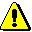This lab requires the use of a DC power supply. The lab supervisor will review the bench supply configuration before the start of the lab. Keep the power OFF while you are wiring your circuit and when you are making changes to your circuit.
1. #### Basic Voltage Measurement

Measure the DC voltage of the 5V bench supply.
2. Measure the DC voltage of the 7.2V battery.
3. Now connect one terminal of the meter to the positive terminal of the 7.2V battery, and the other terminal of the meter to the GROUND of the bench supply. What voltage do you read? What is going on?
4. Now add a connection between the negative terminal of the battery and the GROUND of the bench supply. What voltage do you read?
Explain what is going on to the lab instructor.

5. #### Resistors in Series and Parallel

Construct a circuit with two resistors in series:
• Take two 11 kΩ resistors and measure their actual resistance.
• Construct the following circuit, R1 = R2 = 11 kΩ. Note that this circuit can be drawn two different ways (shown below).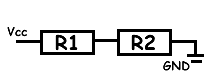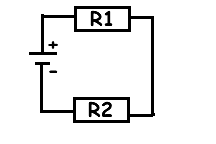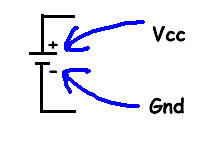• Before connecting power, measure the resistance across the two resistors together.
• Connect power, Vcc = 12 volts, and ground using the bench supplies, and then turn on the power. If you have done this right, nothing exciting should happen. If something exciting happens, e.g. smoke or burning smell, immediately turn off the power and get help from the lab staff.

6. Measure the voltage across each resistor and the two resistors in combination:
• As shown in the diagram below, measure the voltage across R1. What happens if you reverse your voltmeter leads - place red probe where the black probe was and the black probe where the red probe was? What is the relationship between your probe position, the measured value, and the power and ground locations in the circuit?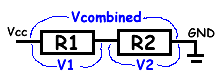• Measure the voltage across R2.
• Measure the voltage across R1 and R2.
• What is the relationship between the Vcombined and the individual voltages? Was your probe placement consistent across all measurements?

7. Using Ohm's Law, calculate the currents I1, I2, and Icombined.

Demonstrate your skill at measuring voltages to the lab instructor.

8. #### Current Measurement

Now you are going to measure the current flowing through each resistor.Always measure current IN SERIES with the load. NEVER measure current across a voltage source.
• Note carefully, that a current measurement requires that the meter be in series with the load. In other words, to measure current the meter must be part of the circuit. Make sure power is off when you are modifying the circuit.
• Note also, that when measuring current, the red lead is connected differently at the meter as compared to the other measurements (resistance, voltage, connectivity)..
• Improper use of the meter when measuring DC current can destroy the meter ... hence all the cautions and notes.

• If you are not sure that you have understood the instructions, have the lab staff check your hook-up before you power up the circuit for the first measurement.
• You have to change the circuit to place the meter in series with the load. Does it matter if the meter is before or after the resistor (the load)? Test this by measuring the current at the three locations shown in the diagram below. Notation: the circle with an A inside is the symbol for an ammeter.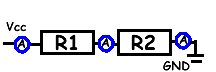If you wire the circuit as in the following diagram, then the ammeter can replace each wire in sequence for each of the measurements.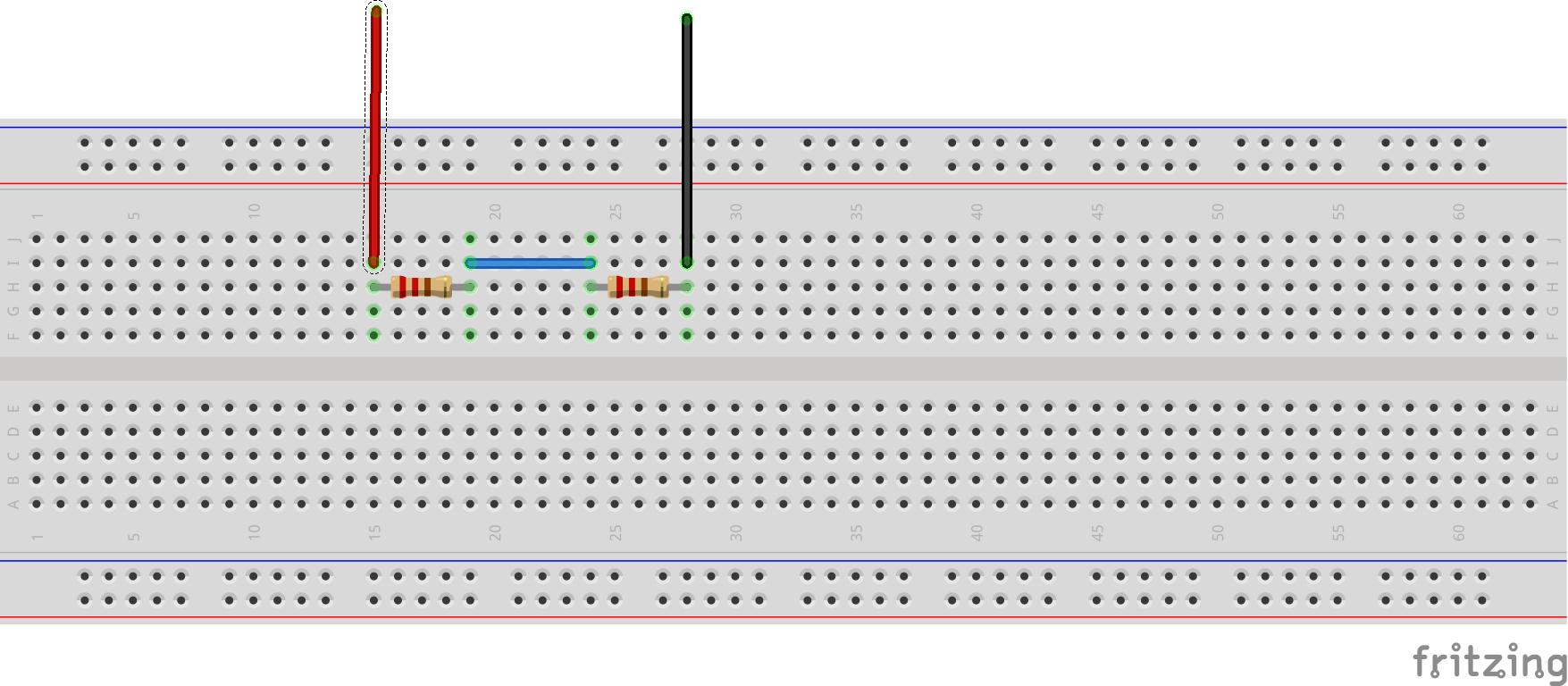Understand that you will modify the circuit three times to take the three measurements.
• How do the measurements compare to the calculations you did in the previous step?
• What did you learn about the currents in a series circuit?

Demonstrate your skill at measuring current to the lab instructor.

9. #### Series and Parallel circuits

For the two resistor series circuit, change R2 so that R2 = 2 x R1 = 20 kΩ (approximation is OK). Measure the actual resistance of R2 before building the circuit. Measure the voltage across each resistor and the resistors in combination. Calculate or measure the currents through each resistor and the resistors in combination. [You notice that I prefer to measure voltage and calculate current. Why?]

10. Modify the series resistor circuit, so that R2 = 10 x R1 = 100 kΩ (approximation is OK). Measure the actual resistance of R2. Measure the voltage across each resistor and the resistors in combination. Calculate or measure the currents through each resistor and the resistors in combination.

When you have resistors in series, does the larger or smaller resistor dominate the circuit?

Does this relationship hold for three resistors as shown below? How would you test this? Demonstrate to lab instructor.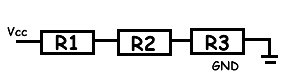11. Construct a circuit with two resistors in parallel as shown below. Let R1 = R2.
• Measure the voltage across R1, across R2, and across R1 in combination with R2. Remember, take one measurement at a time.
• What is the relationship between the individual resistances and the total resistance?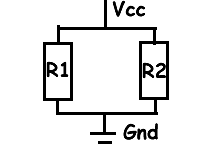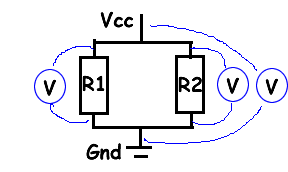• Measure the current through R1, through R2, and through R1 in combination with R2 as shown in the diagram below. Remember, take one measurement at a time.
• What is the relationship between the individual currents and the total current?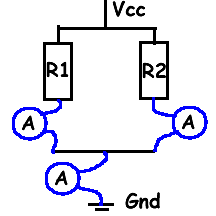If you wire the circuit as in the following diagram, then the ammeter can replace each black wire in sequence for each of the measurements.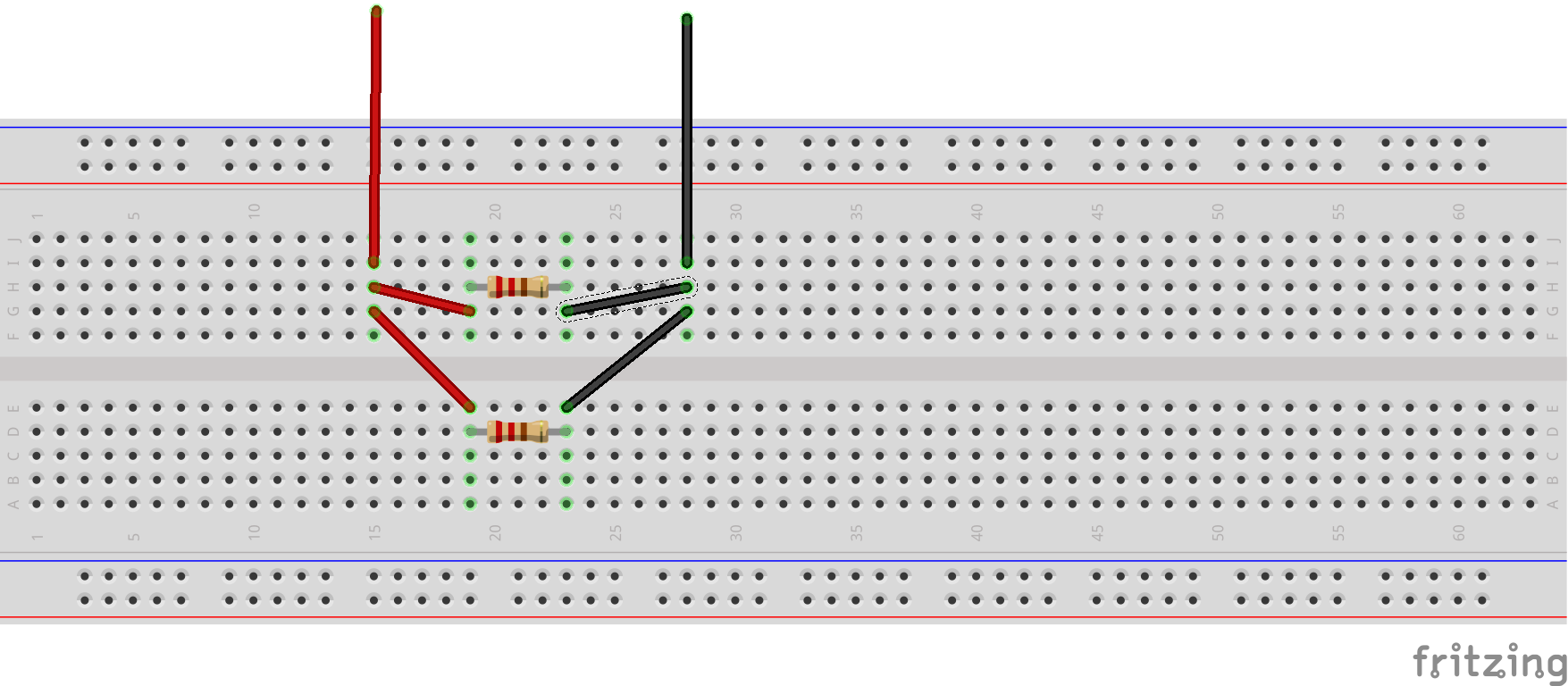12. Repeat your measurements for R2 = 2 x R1 and for R2 = 10 x R1. When you have resistors in parallel, does the larger or smaller resistor dominate the circuit?

Does this relationship hold for three resistors in parallel? How would you test this? Demonstrate to lab staff.

13. Self check: Did you learn anything doing this lab?

• An ammeter (current meter) is always installed in series with a load in the circuit. Since you don't want the measurement to affect the operation of the circuit, should the ammeter behave like an open circuit or a wire (a short)? Does the ammeter have a high internal resistance or a low internal resistance? Explain.

• A voltmeter is always used in parallel with a load in the circuit. Since you don't want the measurement to affect the operation of the circuit, should the voltmeter behave like an open circuit or a wire? Does the voltmeter have a high internal resistance or a low internal resistance? Explain.

## Resources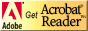If you need to update a browser, you might try Firefox which is
• free
• open source
• available for several platformsSince this page uses cascading style sheets for its layout, it will look best with a browser which supports the specifications as fully as possible.

If you are looking for an office package, with a word processor, spreadsheet, etc., you might try LibreOffice which is
• free
• open source
• available for several platforms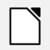Go to the main page for the Department of Physics and Computer Science.

Wilfrid Laurier University